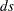The STDIZE Procedure
 Standardization Methods

The following table lists standardization methods and their corresponding location and scale measures available with the METHOD= option.

Table 82.2 Available Standardization Methods

Method

Location

Scale

MEAN

mean

1

MEDIAN

median

1

SUM

0

sum

EUCLEN

0

Euclidean length

USTD

0

STD

mean

standard deviation

RANGE

minimum

range

MIDRANGE

midrange

range/2

MAXABS

0

maximum absolute value

IQR

median

interquartile range

median

median absolute deviation from median

ABW()

biweight 1-step M-estimate

biweight A-estimate

AHUBER()

Huber 1-step M-estimate

Huber A-estimate

AWAVE()

Wave 1-step M-estimate

Wave A-estimate

AGK()

mean

AGK estimate (ACECLUS)

SPACING()

mid-minimum spacing

minimum spacing

L()

L()

L()

IN()

For METHOD=ABW(), METHOD=AHUBER(), or METHOD=AWAVE(),is a positive numeric tuning constant.

For METHOD=AGK(),is a numeric constant giving the proportion of pairs to be included in the estimation of the within-cluster variances.

For METHOD=SPACING(),is a numeric constant giving the proportion of data to be contained in the spacing.

For METHOD=L(),is a numeric constant greater than or equal to 1 specifying the power to which differences are to be raised in computing an L() or Minkowski metric.

For METHOD=IN(),is the name of a SAS data set that meets either of the following two conditions:

• contains a _TYPE_ variable. The observation that contains the location measure corresponds to the value _TYPE_= ’LOCATION’, and the observation that contains the scale measure corresponds to the value _TYPE_= ’SCALE’. You can also use a data set created by the OUTSTAT= option from another PROC STDIZE statement as thedata set. See the section Output Data Sets for the contents of the OUTSTAT= data set.

• contains the location and scale variables specified by the LOCATION and SCALE statements

PROC STDIZE reads in the location and scale variables in thedata set by first looking for the _TYPE_ variable in thedata set. If it finds this variable, PROC STDIZE continues to search for all variables specified in the VAR statement. If it does not find the _TYPE_ variable, PROC STDIZE searches for the location variables specified in the LOCATION statement and the scale variables specified in the SCALE statement.

The variable _TYPE_ can also contain the optional observations, ‘ADD’ and ‘MULT’. If these observations are found in thedata set, the values in the observation of _TYPE_ = ’MULT’ are the multiplication constants, and the values in the observation of _TYPE_ = ’ADD’ are the addition constants; otherwise, the constants specified in the ADD= and MULT= options (or their default values) are used.

For robust estimators, refer to Goodall (1983) and Iglewicz (1983). The MAD method has the highest breakdown point (50%), but it is somewhat inefficient. The ABW, AHUBER, and AWAVE methods provide a good compromise between breakdown and efficiency. The L() location estimates are increasingly robust asdrops from 2 (corresponding to least squares, or mean estimation) to 1 (corresponding to least absolute value, or median estimation). However, the L() scale estimates are not robust.

The SPACING method is robust to both outliers and clustering (Jannsen et al.; 1995) and is, therefore, a good choice for cluster analysis or nonparametric density estimation. The mid-minimum spacing method estimates the mode for small. The AGK method is also robust to clustering and more efficient than the SPACING method, but it is not as robust to outliers and takes longer to compute. If you expectclusters, the argument to METHOD=SPACING or METHOD=AGK should beor less. The AGK method is less biased than the SPACING method for small samples. As a general guide, it is reasonable to use AGK for samples of size 100 or less and SPACING for samples of size 1000 or more, with the treatment of intermediate sample sizes depending on the available computer resources.Previous Page | Next Page | Top of Page